Most Affordable JEE | NEET | 8,9,10 Preparation by Kota's Top IITian Doctor Faculties

# Permutation & Combination - JEE Advanced Previous Year Questions with Solutions`
JEE Advanced Previous Year Questions of Math with Solutions are available at eSaral. Practicing JEE Advanced Previous Year Papers Questions of mathematics will help the JEE aspirants in realizing the question pattern as well as help in analyzing weak & strong areas. eSaral helps the students in clearing and understanding each topic in a better way. eSaral also provides complete chapter-wise notes of Class 11th and 12th both for all subjects. Besides this, eSaral also offers NCERT Solutions, Previous year questions for JEE Main and Advance, Practice questions, Test Series for JEE Main, JEE Advanced and NEET, Important questions of Physics, Chemistry, Math, and Biology and many more. Download eSaral app for free study material and video tutorials.
Q. The number of seven digit integers, with sum of the digits equal to 10 and formed by using the digits 1, 2 and 3 only, is (A) 55            (B) 66           (C) 77               (D) 88 [JEE 2009, 3]
Ans. (C) For sum to be 10 Case-I $\quad 1,2,3,1,1,1,1,=\frac{7 !}{5 !}$ Case-II $\quad 2,2,2,1,1,1,1,=\frac{7 !}{3 ! 4 !}$ So total number $=77$
Q. Let S = {1,2,3,4}. The total number of unordered pairs of disjoint subsets of S is equal to – (A) 25           (B) 34           (C) 42             (D) 41 [JEE 10, 5M, –2M]
Ans. (D) Case- $\mathrm{I}:$ The number of elements in the pairs $(\mathrm{A}, \mathrm{B})$ can be $(1,1) ;(1,2) ;(1,3) ;(2,2)$ $=^{4} \mathrm{C}_{2}+^{4} \mathrm{C}_{1} \times^{3} \mathrm{C}_{2}+^{4} \mathrm{C}_{1} \times^{3} \mathrm{C}_{3}+\frac{^{4} \mathrm{C}_{2} \cdot^{2} \mathrm{C}_{2}}{2}=25$ Case- $\mathrm{II}:$ Number of pairs with $\phi$ as one of subsets $=2^{4}=16$ $\therefore$ Total pairs $=25+16=41$
Q. The total number of ways in which 5 balls of different colours can be distributed among 3 persons so that each person gets at least one ball is – (A) 75           (B) 150           (C) 210              (D) 243 [JEE 2012, 3M, –1M]
Ans. (B) Balls can be distributed as $1,1,3$ or $1,2,2$ to each person. When $1,1,3$ balls are distributed to each person, then total number of ways: $=\frac{5 !}{1 ! 1 ! 3 !} \cdot \frac{1}{2 !} 3 !=60$ When $1,2,2$ balls are distributed to each person, then total number of ways : $=\frac{5 !}{1 ! 2 ! 2 !} \cdot \frac{1}{2 !} 3 !=90$ $\therefore$ total $=60+90=150$
Let $\mathrm{a}_{\mathrm{n}}$ denotes the number of all n-digit positive integers formed by the digits 0, 1 or both such that no consecutive digits in them are 0. Let $b_{n}$ = the number of such n-digit integers ending with digit 1 and $\mathrm{C}_{\mathrm{n}}$ = the number of such n-digit integers ending with digit 0.
Q. The value of $\mathrm{b}_{6}$ is (A) 7            (B) 8          (C) 9             (D) 11 [JEE 2012, 3M, –1M]
Ans. (B) $\mathrm{a}_{1}=1, \mathrm{a}_{2}=2$ So $a_{3}=3, \quad a_{4}=5 a_{5}=8$ $\Rightarrow b_{6}=a_{5}=8$
Q. Which of the following is correct ? (A) $a_{17}=a_{16}+a_{15}$ (B) $\mathrm{c}_{17} \neq \mathrm{c}_{16}+\mathrm{c}_{15}$ (C) $\mathrm{b}_{17} \neq \mathrm{b}_{16}+\mathrm{c}_{16}$ (D) $a_{17}=c_{17}+b_{16}$ [JEE 2012, 3M, –1M]
Ans. (A) $a_{n}=a_{n-1}+a_{n-2}$ put $n=17$ $a_{17}=a_{16}+a_{15} \quad($ A) is correct $c_{n}=c_{n-1}+c_{n-2}$ $\mathrm{c}_{\mathrm{n}}=\mathrm{c}_{\mathrm{n}-1}+\mathrm{c}_{\mathrm{n}-2}$ $\mathrm{So}$ put $\mathrm{n}=17$ $\mathrm{c}_{17}=\mathrm{c}_{16}+\mathrm{c}_{15} \quad(\mathrm{B})$ is incorrect $\mathrm{b}_{\mathrm{n}}=\mathrm{b}_{\mathrm{n}-1}+\mathrm{b}_{\mathrm{n}-2}$ put $\mathrm{n}=17$ $\mathrm{b}_{17}=\mathrm{b}_{16}+\mathrm{b}_{15} \quad(\mathrm{C})$ is incorrect $\mathrm{a}_{17}=\mathrm{a}_{16}+\mathrm{a}_{15}$ while $(\mathrm{D})$ says $\mathrm{a}_{17}=\mathrm{a}_{15}+\mathrm{a}_{15} \quad$ (D) is incorrect
Q. Let $n_{1} [JEE(Advanced)-2014, 3] Ans. 5 as$n_{1} \geq 1, n_{2} \geq 2, n_{3} \geq 3, n_{4} \geq 4, n_{5} \geq 5$Let$n_{1}-1=x_{1} \geq 0, n_{2}-2=x_{2} \geq 0 \ldots \ldots \ldots \ldots n_{5}-5=x_{5} \geq 0\Rightarrow$New equation will be$\quad x_{1}+1+x_{2}+2+\ldots \ldots x_{5}+5=20\Rightarrow x_{1}+x_{2}+x_{3}+x_{4}+x_{5}=20-15=5$Now$x_{1} \leq x_{2} \leq x_{3} \leq x_{4} \leq x_{5}$AND Numbr of red line segments$=^{\mathrm{n}} \mathrm{C}_{2}-\mathrm{n}$Number of blue line segments$=\mathrm{n}\therefore^{\mathrm{n}} \mathrm{C}_{2}-\mathrm{n}=\mathrm{n}\frac{\mathrm{n}(\mathrm{n}-1)}{2}=2 \mathrm{n} \Rightarrow \mathrm{n}=5$Ans. Q. Let n$\geq$2 b an integer. Take n distinct points on a circle and join each pair of points by a line segment. Colour the line segment joining every pair of adjacent points by blue and the rest by red. If the number of red and blue line segments are equal, then the value of n is [JEE(Advanced)-2014, 3] Ans. 7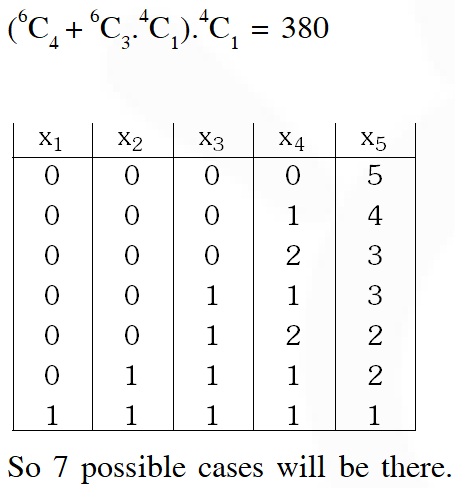Q. Six cards and six envelopes are numbered 1, 2, 3, 4, 5, 6 and cards are to be placed in envelopes so that each envelope contains exactly one card and no card is placed in the envelope bearing the same number and moreover the card numbered 1 in always placed in envelope numbered 2. Then the number of ways it can be done is – (A) 264 (B) 265 (C) 53 (D) 67 [JEE(Advanced)-2014, 3(–1)] Ans. (C) Total number of dearrangement 6!$\left[\frac{1}{2 !}-\frac{1}{3 !}+\frac{1}{4 !}-\frac{1}{5 !}+\frac{1}{6 !}\right]=360-120+30-6+1=240+25=265$There are equal chances that card 1 goes into any envelope from 2 to$6 \quad \therefore \quad \frac{1}{5}(265)=53$Q. Let n be the number of ways in which 5 boys and 5 girls can stand in a queue in such a way that all the girls stand consecutively in the queue. Let m be the number of ways in which 5 boys and 5 girls can stand in a queue in such a way that exactly four girls stand consecutively in the queue. Then the value of$\frac{\mathrm{m}}{\mathrm{n}}$is [JEE (Advanced) 2015, 4M, –0M] Ans. 5$n=6 ! \times 5 !n=6 ! \times 4 ! \times 5 \times^{5} C_{4}\frac{m}{n}=\frac{6 ! \times 4 ! \times 5 \times^{5} C_{4}}{6 ! \times 5 !}=5$Q. A debate club consists of 6 girls and 4 body. A team of 4 members is to be selected from this club including the selection of a captain (from among these 4 member) for the team. If the team has to include at most one boy, then the number of ways of selecting the team is (A) 380 (B) 320 (C) 260 (D) 95 [JEE (Advanced) 2016] Ans. (A) Q. Words of length 10 are formed using the letters A, B, C, D, E, F, G, H, I, J. Let x be the number of such words where no letter is repeated; and let y be the number of such words where exactly one letter is repeated twice and no other letter is repeated. Then,$\frac{\mathrm{y}}{9 \mathrm{x}}$= [JEE (Advanced) 2017] Ans. 5$\mathrm{x}=10 !\mathrm{y}=^{10} \mathrm{C}_{1}^{9} \mathrm{C}_{8} \frac{10 !}{2 !}$Q. Let$S=\{1,2,3, \ldots \ldots, 9\} .$For$\mathrm{k}=1,2, \ldots . ., 5,$let$\mathrm{N}_{\mathrm{k}}$be the number of subsets of$\mathrm{S},$each containing five elements out of which exactly$\mathrm{k}$are odd. Then$\mathrm{N}_{1}+\mathrm{N}_{2}+\mathrm{N}_{3}+\mathrm{N}_{4}+\mathrm{N}_{5}=$b (A) 125 (B) 252 (C) 210 (D) 126 [JEE (Advanced) 2017] Ans. (D)$\mathrm{N}_{1}+\mathrm{N}_{2}+\mathrm{N}_{3}+\mathrm{N}_{4}+\mathrm{N}_{5}=$Total ways$-\{\text { when no odd }\}$Total ways$=^{9} \mathrm{C}_{5}$Number of ways when no odd, is zero$\quad(\because \text { only available even are } 2,4,6,8)\therefore$Ans$:^{9} \mathrm{C}_{5}-$zero$=126$Q. The number of 5 digit numbers which are divisible by 4, with digits from the set {1, 2, 3, 4, 5} and the repetition of digits is allowed, is —— [JEE (Advanced) 2018,3(0)] Ans. 625 Option for last two digits are$(12),(24),(32),(44)$are$(52) .\therefore \quad$Total No. of digits$=5 \times 5 \times 5 \times 5=625$Q. In a high school, a committee has to be formed from a group of 6 boys$\mathrm{M}_{1}, \mathrm{M}_{2}, \mathrm{M}_{3}, \mathrm{M}_{4}, \mathrm{M}_{5},\mathrm{M}_{6}$and 5 girls$\mathrm{G}_{1}, \mathrm{G}_{2}, \mathrm{G}_{3}, \mathrm{G}_{4}, \mathrm{G}_{5}$(i) Let$\alpha_{1}$be the total number of ways in which the committee can be formed such that the committee has 5 members, having exactly 3 body and 2 girls. (ii) Let$\alpha_{2}$be the total number of ways in which the committe can be formed such that the committee has at least 2 members, and having an equal number of boys and girls. (iii) Let$\alpha_{3}$be the total number of ways in which the committe can be formed such that the committee has 5 members, at least 2 of them being girls. (iv) Let$\alpha_{4}$be the total number of ways in which the committee can be formed such that the commitee has 4 members, having at least 2 girls and such that both$\mathrm{M}_{1}$and$\mathrm{G}_{1}\$ are NOT in the committee together.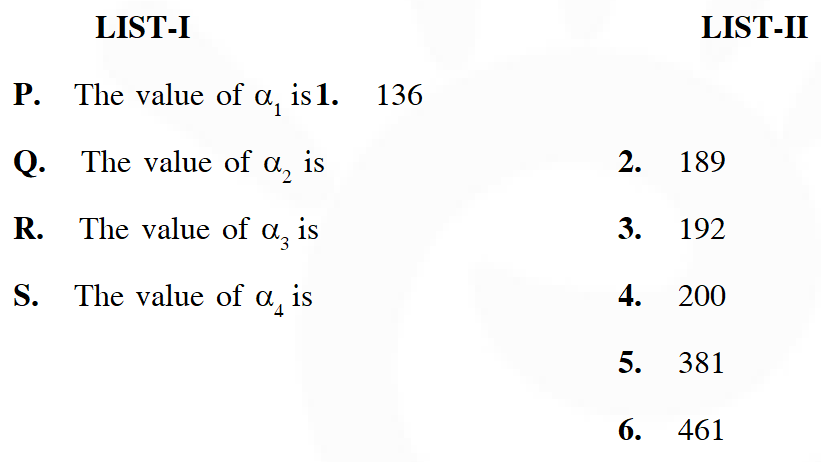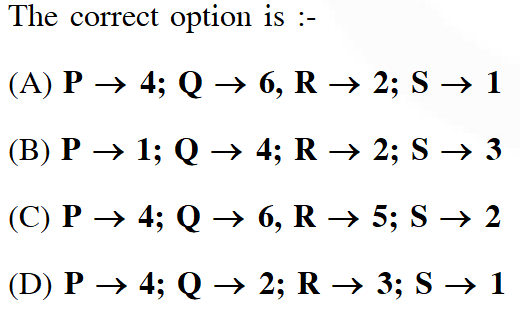[JEE (Advanced) 2018,3(–1)]
Ans. (C)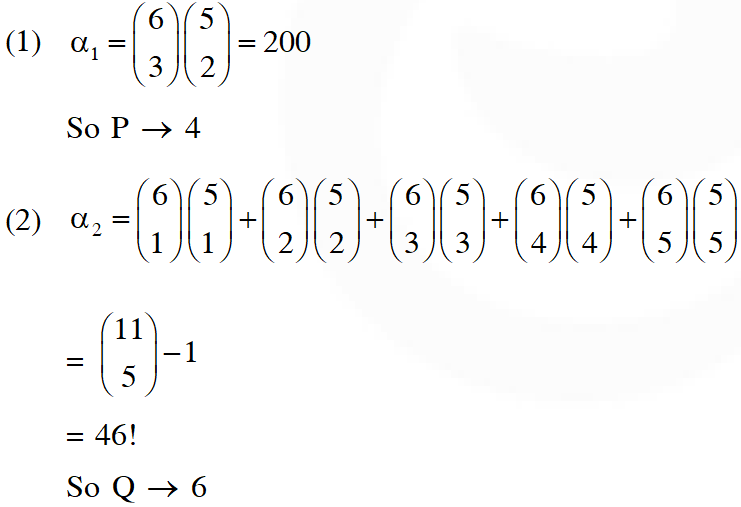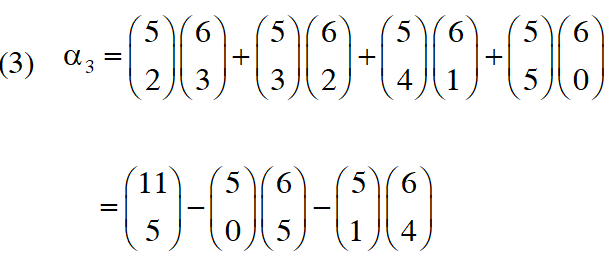Vaibhav Samdani
Sept. 25, 2022, 9:44 a.m.
Last Question me JEE Advanced 2018 ka solution pura nahi h!
john
Aug. 19, 2022, 12:32 p.m.
good
.
Aug. 19, 2022, 12:32 p.m.
.
Just trying to help
Aug. 19, 2022, 12:31 p.m.
Five persons A,B,C,D and E are seated in a circular arrangement .If each of them is given a hat of one of the three colours red, blue and green ,then the numbers of ways of distributing the hats such that the person seated in adjacent seats get different coloured hats is___ [JEE ADVANCED 2019]
Just Helping
Aug. 19, 2022, 12:29 p.m.
Five persons A,B,C,D and E are seated in a circular arrangement .If each of them is given a hat of one of the three colours red, blue and green ,then the numbers of ways of distributing the hats such that the person seated in adjacent seats get different coloured hats is___ [JEE ADVANCED 2019]
Ananya
Aug. 19, 2022, 12:16 p.m.
Very helpful as i found all ques here for practice but the answers of some ques are wrong.. Still did good for me.
MUSKAAN MEHROTRA
Jan. 14, 2021, 1:47 p.m.
I THINK ANSWER TO QUESTION 3 FROM THE TOP IS WRONG... IT SHOULD BE 243-3=240 BECAUSE BALLS ARE DISTINCT SO IT SHOULD BE( 3^(5))-3
thor
Dec. 31, 2020, 9:05 a.m.
which ans is wrong?
Dec. 19, 2020, 8:27 p.m.
literally stupid
aa
Dec. 18, 2020, 7:34 p.m.
crap
Anonymous
Oct. 15, 2020, 11:21 p.m.
Many of the answers are wrong and few questions are incomplete.
Dhruvil
Sept. 15, 2020, 2:52 p.m.
Thanks you for providing this material 🙌🙏
its confidential
Sept. 5, 2020, 9:57 p.m.
thank you sir its help me a lot
its confidential
Sept. 5, 2020, 9:57 p.m.
thank you
Pavithra
May 20, 2020, 7:20 p.m.
Help full